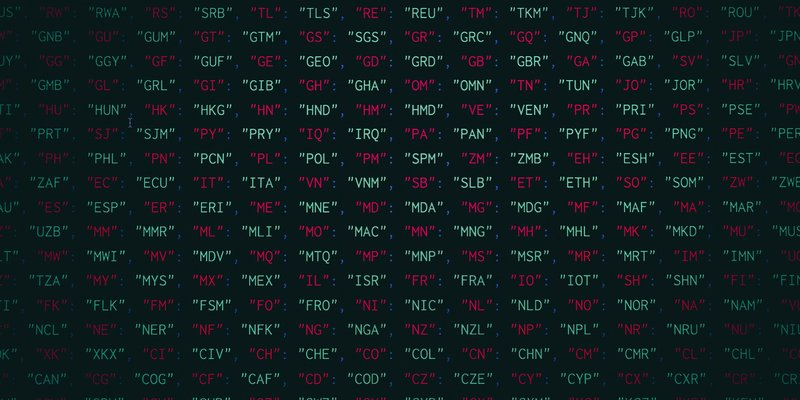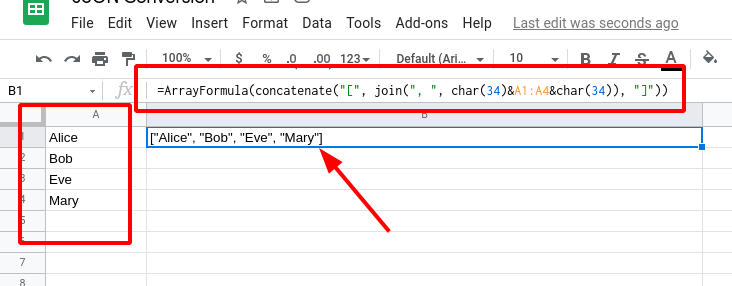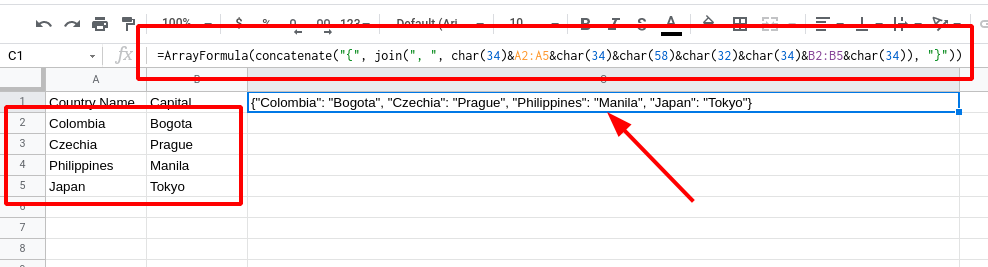## Google Sheets Tip: Generate JSON Data from Rows and ColumnsYou may encounter a scenario where you need to convert data from your Google Sheets spreadsheet to JSON format.  You may need this to use as input to another app or system.

For example, our EZ Exporter app supports a function that takes a raw JSON string that can be used for looking up values (like a lookup table).

JSON data can have complex nested structure but a typical format you'll see is an array that looks like this:

`["Alice", "Bob", "Eve", "Mary"]`

Or a key/value (i.e. dictionary) format that looks something like this:

`{"Colombia": "Bogota", "Czechia": "Prague", "Philippines": "Manila", "Japan": "Tokyo"}`

You can generate JSON-formatted data like these in Google Sheets using a formula.

Let's start with the simpler example to convert a range of rows in one column into a simple JSON array:The formula used in the example above is the following:

`=ArrayFormula(concatenate("[", join(", ", char(34)&A1:A4&char(34)), "]"))`

It first uses the ArrayFormula function to apply a formula for each row in the selected range (from A1 to A4).  For the value in each row, we enclose them with quotes (i.e. the char(34) part in the formula) and then join them together.  After that, we then add the opening and closing square brackets.

Now let's do a more complex example involving two columns where the first column will be the "key" and the second column is the "value".  In the example below, the first column is the country and the second column is the country's capital.The formula:

`=ArrayFormula(concatenate("{", join(", ", char(34)&A2:A5&char(34)&char(58)&char(32)&char(34)&B2:B5&char(34)), "}"))`

The formula looks very complex but it's actually very similar to the first one. We're just using two separate ranges of cells here and replacing the square braces with curly braces.  The parts with the char() are the unicode representations of different characters:

• char(34) - double quote
• char(58) - colon
• char(32) - space

To summarize, the main Google Sheets function to use here is the ArrayFormula() function. We then combine the data for each element in the array using the join() function and then use concatenate() to add the opening and closing braces.

We hope this little tutorial proves useful!